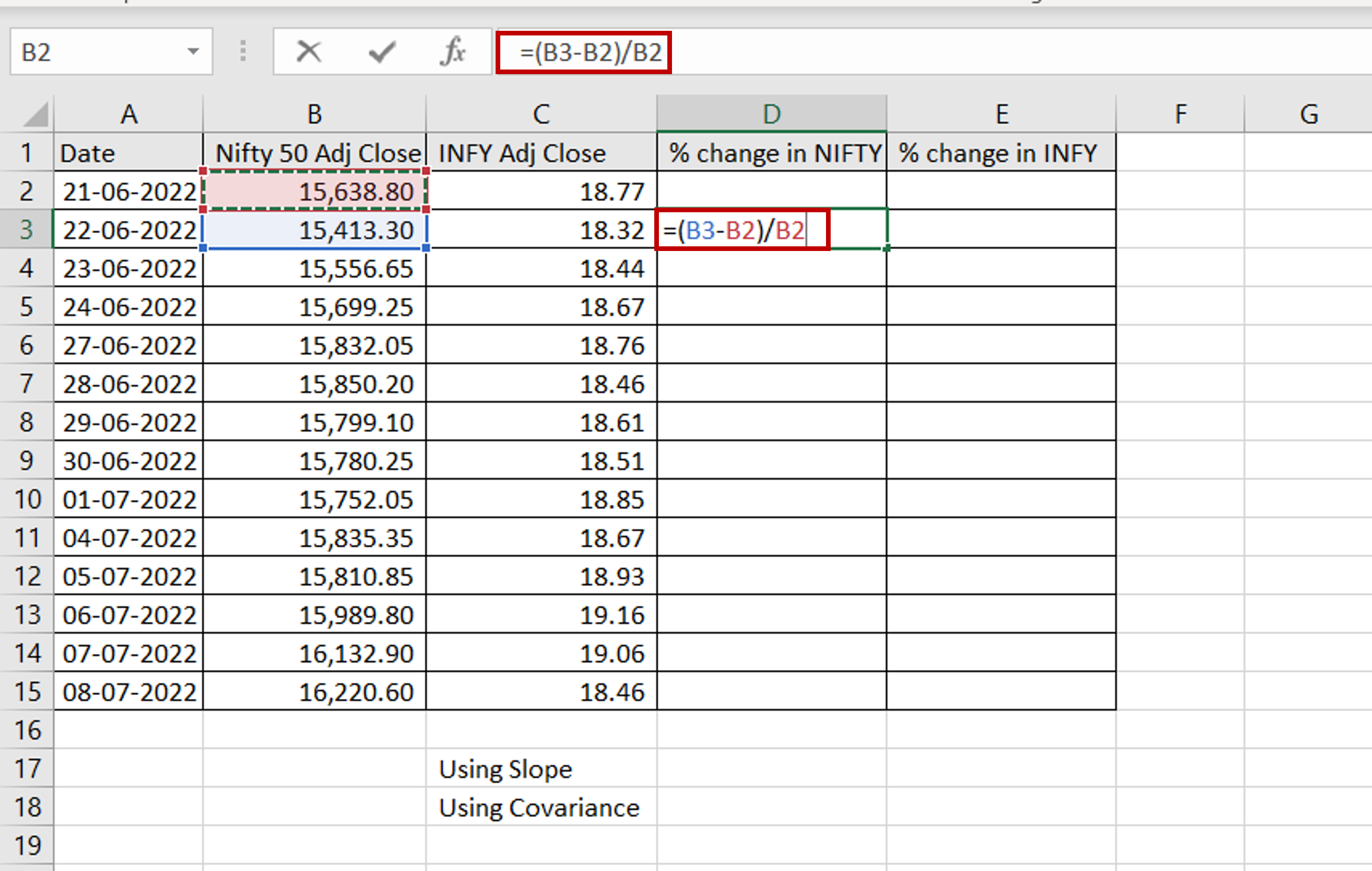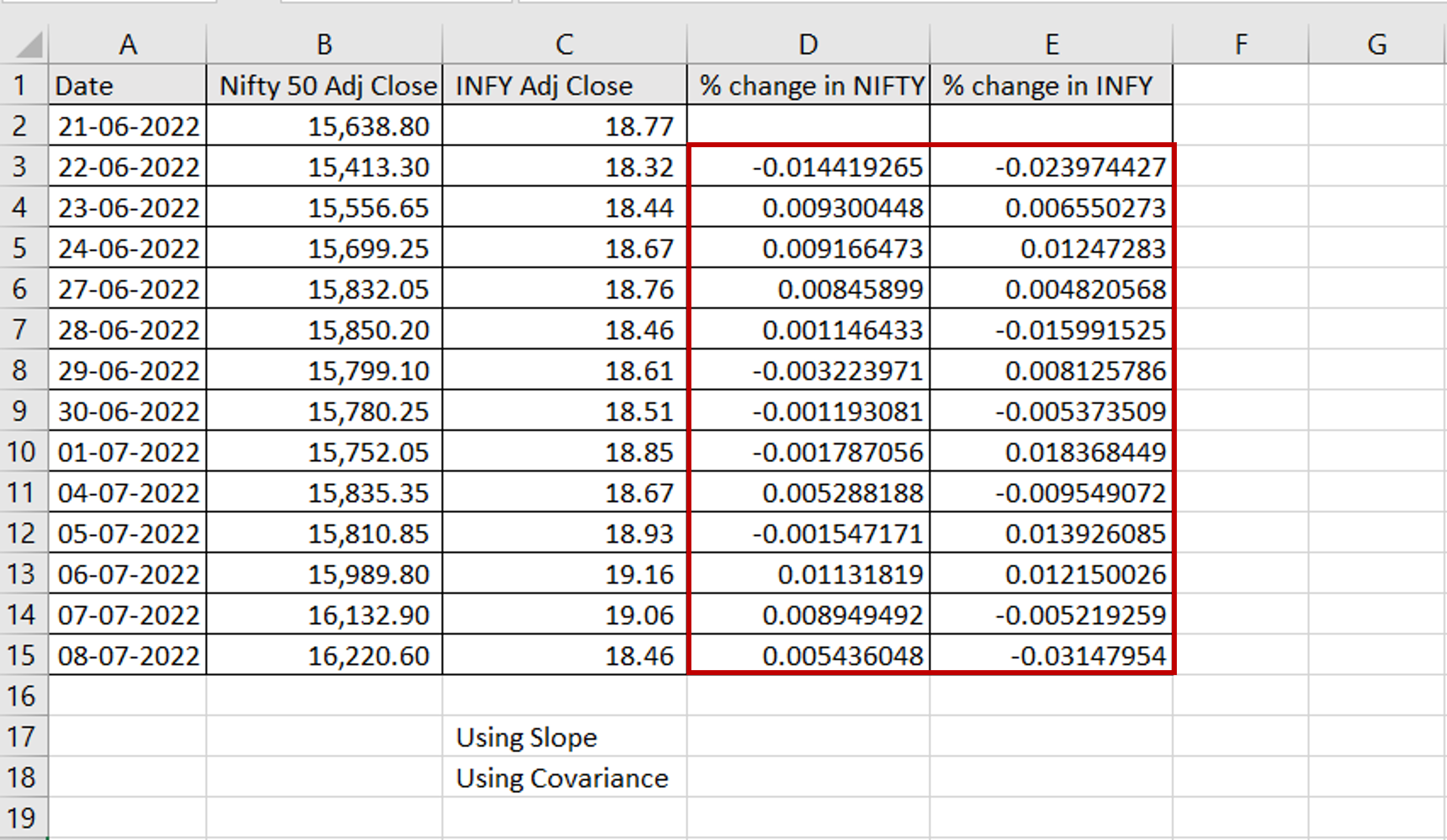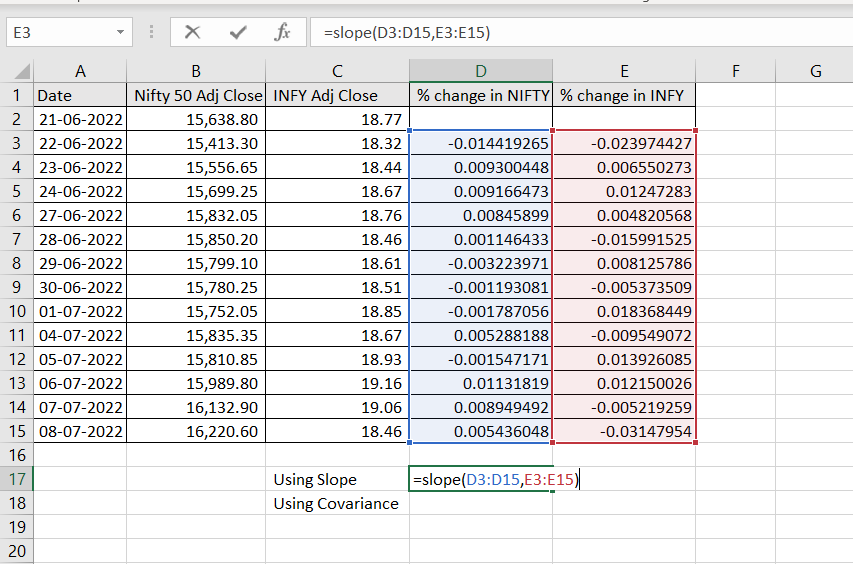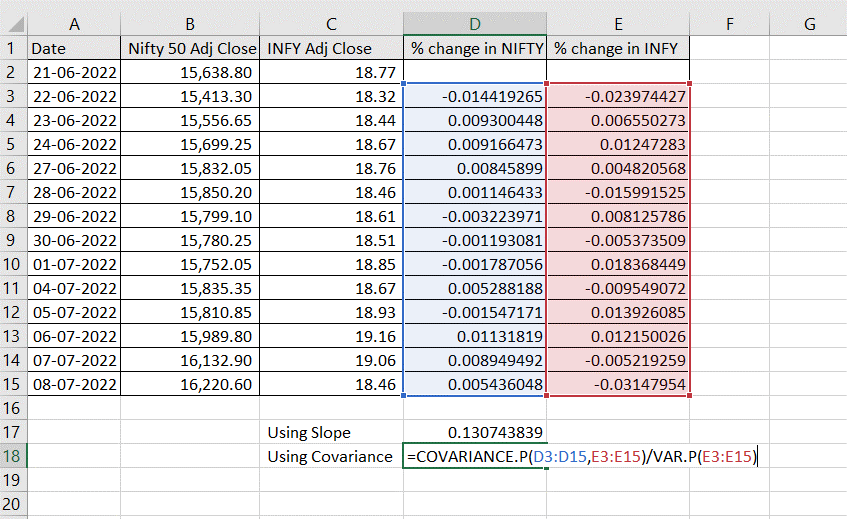# How to calculate beta in Excel

You can watch a video tutorial here.Beta is a very important metric for financial analysts. It measures the volatility of a stock (or a portfolio of stocks) when compared to the rest of the stock market. In Excel, you can calculate the Beta of a stock using either the Covariance and Variance functions or the Slope function. Here we use both methods.

### Step 1 – Compute the percentage change in price– In the second row of the ‘% change in NIFTY’ column, enter the formula for the change in price:
=(<cell reference of the ‘Nifty 50 Adj Close’ column> –
<cell reference of the previous ‘Nifty 50 Adj Close’ column>) /
<cell reference of the ‘Nifty 50 Adj Close’ column >

### Step 2 – Copy the formula– Select the cell with the formula and press Ctrl+C or choose Copy from the context menu (right-click)
– Select the rest of the cells in the column and in the ‘% change in INFY’ column and press Ctrl+V or choose Paste from the context menu (right-click)

### Step 3 – Use the SLOPE() function– Enter the formula for the slope:
=slope(<range of the ‘% change in NIFTY’ column>,
<range of the ‘% change in INFY’ column>)

### Step 4 – Use the Covariance formula– Enter the formula for the covariance:
=covariance.p(<range of the ‘% change in NIFTY’ column>,
<range of the ‘% change in INFY’ column>)/
variance.p(<range of the ‘% change in INFY’ column>)

### Step 5 – Check the result– Both formulas give the same value which is the Beta for the INFY stock